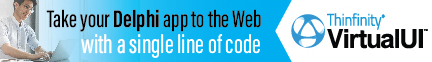DelphiBasicsAbsFunction Gives the absolute value of a number (-ve sign is removed) System unit
 function Abs ( Number : Numeric type ) : Numeric type;
Description
The Abs function returns the absolute value of a negative or positive number. It does this by removing a negative sign, if found.

The Number can be any numeric type, and can even be a Variant, as long as it can be converted to a number. For example, a Variant set to a string '-1.23' will work fine. Always, Abs converts the Variant to an Extended floating point number prior to removing any negative sign, even if the result is an integer value.
Notes
Floating point numbers can be set to extreme values, such as infinity (see the example). The Abs function simply removes the negative sign of these, so that -INF becomes INF.
Related commands
 Div Performs integer division, discarding the remainder Mod Performs integer division, returning the remainderDownload this web site as a Windows program.